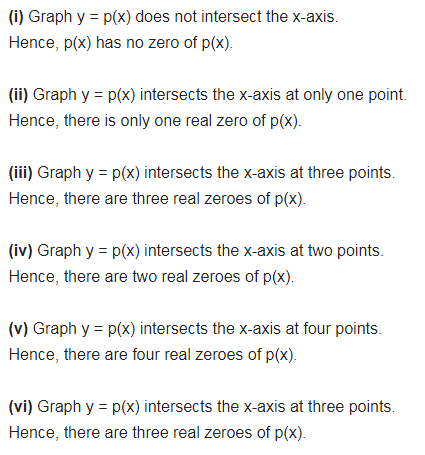# The graphs of y = p(x) are given below for some polynomials p(x)

The graphs of y = p(x) are given below for some polynomials p(x). Find the number of zeroes of p(x) in each case.